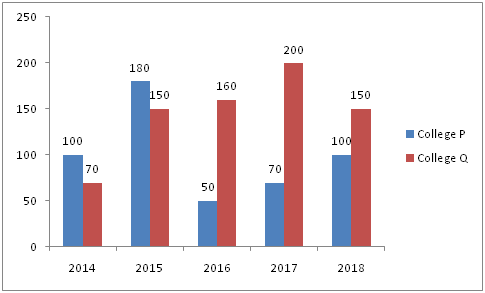# RBI Assistant Prelims Quantitative Aptitude Questions 2019-20 – (Day-01)

Dear Aspirants, Our IBPS Guide team is providing new series of Quantitative Aptitude Questions for RBI Assistant Prelims 2019-20 so the aspirants can practice it on a daily basis. These questions are framed by our skilled experts after understanding your needs thoroughly. Aspirants can practice these new series questions daily to familiarize with the exact exam pattern and make your preparation effective.

Ensure Your Ability Before of Exam

Take RBI Assistant Prelims Free Mock test

[WpProQuiz 7545]

Data Interpretation

Directions (1 – 5): Study the following information carefully and answer the questions given below.

The below graph shows the total number of students placed in campus interview from two colleges P and Q in five different years.1) If the respective ratio of total number of students placed in campus interview from colleges P and Q together in 2017 and 2019 is 5: 7, what was the total number of students placed from the colleges P and Q together in 2018?

a) 456

b) 530

c) 378

d) 560

e) None of these

2) In 2016, 40% of students placed in campus interview from college P and 30% of students placed in campus interview from college Q were females. What was the total number of female students placed in campus interview from colleges P and Q together in 2016?

a) 62

b) 64

c) 66

d) 68

e) None of these

3) Number of students placed in campus interview from college P increased by what percent from 2014 to 2015?

a) 40%

b) 80%

c) 85%

d) 70%

e) None of these

4) What is the average number of students placed in campus interview from college Q in 2014, 2015, 2016, 2017 and 2018?

a) 146

b) 141

c) 123

d) 132

e) None of these

5) In 2015, out of the total number of students placed in campus interview from colleges P and Q together, only 60% of the students accepted the job offer. What was the total number of students from colleges P and Q together accepted the job offer in 2015?

a) 198

b) 190

c) 189

d) 160

e) None of these

Simplifications

Direction (6 – 10): What value will come in place of question mark (?) in the following questions?

6) 162 + 132 + 152 + 112 =?

a) 821

b) 771

c) 681

d) 721

e) 801

7) 43 * 37 + 54 * 46 – 62 * 58 =?

a) 521

b) 703

c) 549

d) 637

e) 479

8) 2.25 * 16 + 5.25 * 8 =? + 43

a) 43

b) 78

c) 61

d) 53

e) 35

9) 15% of 540 + 25% of? = 61% of 200

a) 84

b) 128

c) 156

d) 112

e) 164

10) 144 * 36 – 100 * ? = -2016

a) 84

b) 63

c) 112

d) 72

e) 95

Directions (1-5) :

Total number of students placed in campus interview from colleges P and Q together in 2017 = 70 + 200 = 270

Total number of students placed in campus interview from colleges P and Q together in 2019= 270 * 7/5 = 378

Total number of female students placed in campus interview from colleges P and Q together in 2016 = 40% of 50 + 30% of 160

= 40/100 * 50 + 30/100 * 160

= 20 + 48 = 68

Required percentage = (180 – 100)/100 * 100 = 80%

Required average = (70 + 150 + 160 + 200 + 150)/5 = 146

Total number of students accepted the job offer from the colleges P and Q together in 2015 = 60% of (180 + 150)

= 60/100 * 330 = 198

?= 256 + 169 + 225 + 121 = 771

?= (40 + 3)(40 – 3) + (50 + 4)(50 – 4) – (60 + 2)(60 – 2)

= 402 – 32 + 502 – 42 – 602 + 22

= 1600 – 9 + 2500 – 16 – 3600 + 4

= 479

? + 75 = 36 + 42 = 78

=> ? = 78 – 43 = 35

81 + 25% of  ? = 122

=> 25% of ? = 122 – 81 = 41

=> ? = 41 * 100/25 = 164

144 * 36 – 100 *? =-2016

=> 100 x ? = 144*36 + 2016

= 5184 + 2016 = 7200

=> ? = 72